Showing posts with label Central tendency. Show all posts
Showing posts with label Central tendency. Show all posts

# Measures of central tendency

By
Lubna Toheed
Introduction
Measures of central tendency describe how the data cluster together around a central point. It is useful for making comparisons between groups of individuals or between sets of figures. It reduces a large number of measurements to a single figure, and thus makes comparisons easy.
Unfortunately, there is no single, standard procedure for determining central tendency. The problem is that no single measure will always produce a typical, representative value in every situation. Therefore, we use different ways to measure central tendency. There are three common measures of central tendency:
1.      The Mean or Average or arithmetic Mean,
2.      The median and ,
3.      The mode.
These three statistical measures are computed differently and have different characteristics. Here we will discuss ‘Mean’ and ‘Median’ only.
Mean
The Mean of a distribution is known is “Arithmetic Mean”. Mean indicate the average value of the group. The Mean in each group or condition is calculated by adding up all the scores in a given condition, and then dividing by the number of participants in that condition.  It can be defined as “ the point on the scale of measurement obtained by dividing sum of all scores by the number of the scores.
Uses of Mean
1.       Mean is used when a reliable and accurate measure of central tendency is needed.
2.       Mean is used when the same scores are distributed symmetrically around the central point.
3.      Mean is used when the greatest stability is required
4.      Mean is used when other stastical calculation S.D./ M.D./ A.D. are to be completed.
1. Mean is used when we are having a series with no extreme items.

Calculation Of mean
Mean is calculated by two type of data
(1)    Ungrouped Data
(2)    Grouped Data

(1)Ungrouped Data

We use the following formula to find the mean of ungrouped data: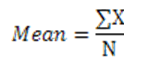Where ,
∑ stand for ‘sum of’ or ‘Sum Total’
X Stand for the individual scores
N stand for total number of scores

Example:

Following are the marks of the students in the subject of mathematics.
 Setudnts marks ABCDE 7030209040 N==5 ∑x = 250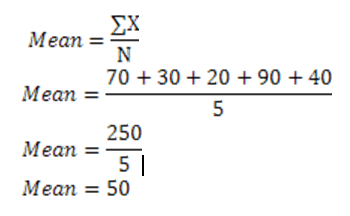(1)   Grouped Data
When the scores are large in number, the data is first grouped into the frequency distribution table and then the mean is calculated by using the following formulas:

a)Long Method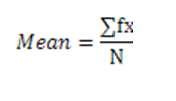where ,
∑ stand for ‘ sum of’ or ‘ Sum Total”
f stand for frequency
x is the mid- point of class interval
N is the total number of scores

b) Short Method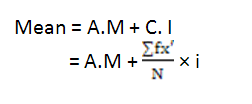Where,
C is correction factor with response to class interval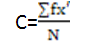A.M = Assumed mean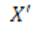= Deviations of mid-point of the class interval from A.M divided by the size of class interval
F = frequency
I = size of the class interval.

Examples:
a)Using Long method
Calculate mean for  the following grouped data:

 s.no Class interval Frequency 12345 10-1415-1920-2425-2930-34 54632 N=20

The above data is consisted of class interval and frequencies. The size of each class interval is 5. It is difficult to know the aggregate of each class interval. So it is necessary to find out the mid- point of each class interval by using the following formula: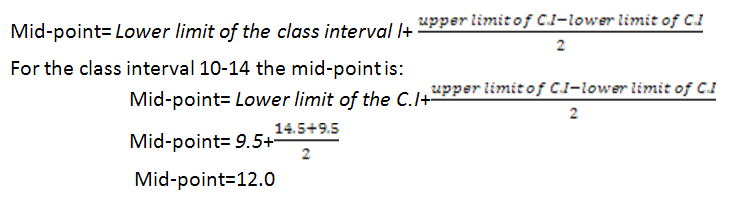In the table below mid-points and fx for each class interval has been calculated

 S.no C.I Class limits f x fx 12345 10-1415-1920-2425-2930-34 9.5-14.514.5-19.519.5-24.524.5-29.529.5-34.5 54632 1217222732 60681328164 N= 20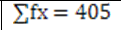Putting values in formula: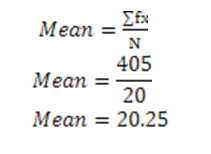b) Using Short Method

Using short method mean is calculated by taking the assumed mean from any class interval.
Suppose assumed mean (A.M) remain in the C.I. 22-24. The mid point of that C.I. is 22, is regarded as A.M.
While calculatingof any class interval the formula used is: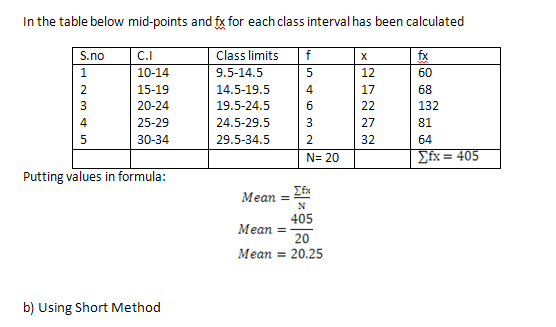Using short method mean is calculated by taking the assumed mean from any class interval.
Suppose assumed mean (A.M) remain in the C.I. 22-24. The mid point of that C.I. is 22, is regarded as A.M.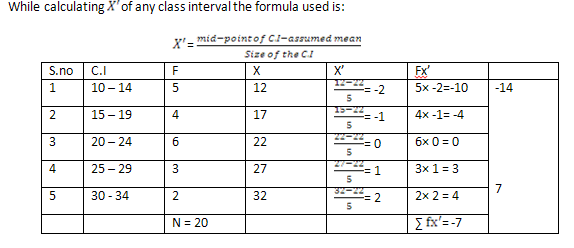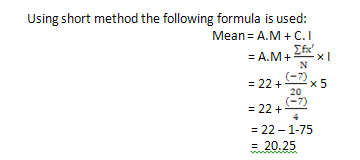Properties of Mean

1.      The sum of deviations of all scores in a set from their Arithmetic Mean is “0”.
2.      The Sum of deviation from Arithmetic Mean is less then the sum of square of deviations from any other value.
3.      If each score of a series is subtracted by a constant quantity, the Mean will be as increased or decreased by the same quantity.
4.      If each score of a series divided or multiplied by a constant quantity, the mean will be multiplied or divided by the same quantity.

Merits of Mean

1.      It is rigidly defined.
2.      It is based on all the values.
3.      It is more stable than any other method of  average.
4.      It is relatively reliable.
5.      It is the center of gravity, balancing the values on either side of it.
6.      It has further algebraic treatment capacity.

Demerits of Mean

1.      It is highly affected by abnormal values.
2.      The loss of even a single observation makes it impossible to compute the aritmetic mean correctly.

Limitations
Mean can be very misleading if the distribution differs markedly from the normal and there are one or two extreme scores in one direction.

Median
The Median is the middle item when the data is listed in order. It is the point below which remain 50% of ‘N’ and above which remain the other 50% of N. So it is also called the 5th percentile. Mean is called the positional average .The position refer to the place of the value in a series.
Median is used:
1. When  mid-point of the given distribution is to be found.
2. When the series contain extreme scores.
3. When there is open end distribution it is more reliable than Mean.
4. Mean cannot be calculated graphically, median can be calculated graphically.
5. For or articles that cannot be precisely measured.

Calculation Of mean

Mean is calculated for two types of data
(a)    Ungrouped Data
(b)    Grouped Data

(a)   Ungrouped Data

(1)    If there is an odd number of scores, then the median is simply the middle score, having an equal number of scores higher and lower than it.
The formula for the calculation of the  ungrouped data: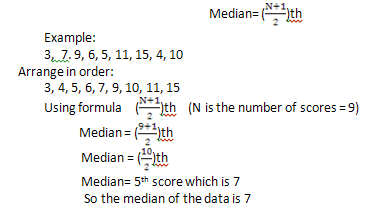(2)  If there is an even number of scores. In that case, we work out the mean of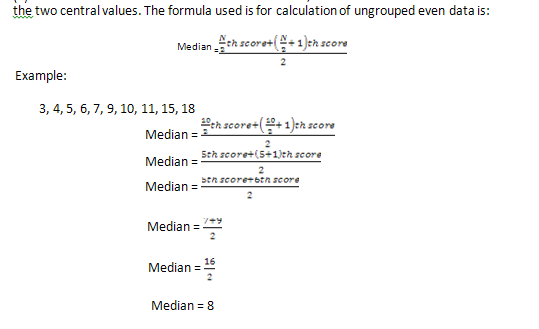(b)  Grouped data
When the score is in a continuous series, it is grouped into the frequency distribution table and the Median is then calculated by using formula: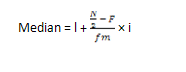Example:
Calculate the Median from the following distribution table.
 C.I. 50-54 55-59 60-64 65-69 70-74 75-79 80-84 85-89 f 8 12 24 16 32 20 11 5

Procedure to calculate median
1.      Locate the Median group  by N/2th
2.      Find out the cumulative frequencies
3.      Point out where N/2th score lies in which class interval
4.      Apply the Formula of Median.

 S.no C.I C.I. f cf 1234 50-5455-5960-6465-69 49.5-54.554.5-59.559.5- 64.564.5- 69.5 8122416 88+12=2020+24=4444+16=60 5 70-74 69.5-74.5 32 60+32=92 678 75-7980-8485-89 74.5-79.579.5- 84.584.5-89.5 20115 92+20=112112+11=123123+5 = 128 N = 128

Where N/2 is 128/2 = 64
64 frequency remains in the class interval 69.5- 74.5 where the cumulative frequency is 92.
The Formula is: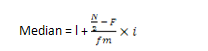l = lower limit of class interval where N/2 lies
N = total number of frequencies
F = Total number of class frequencies of the C.I. above the C.I. where N/2 lies
fm = Actual number of frequencies of C.I. where N/2 lies
i = size of the C.I.
So, l = 69.5
N = 128
F =  60
fm = 32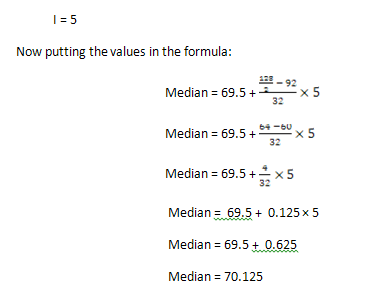Merits of median
1.      It is easily calculated
2.      It is specially used in open-ended class interval
3.      It is not affected by the values of extreme items as it focuses only on scores in the middle of the distribution.
4.      It is the most appropriate average in dealing with qualitative data

Demerits of Median
1.      In calculating Median the data are to be arranged in descending or ascending order
2.      which is time consuming
3.      Since it is a positional average, its value is not determined by each and every observation.
4.      It may sometimes be indefinite, when the number of items in a median class is large.
5.      Median may sometimes be located at the point where the frequency may be quite small.

Limitations
1.      It is not suitable for further algebraic treatment.
2.      It can’t be used for computing other statistical measures such as S.D., co-efficient of correlation.
3.      The main limitation of the median is that it ignores most of the scores, and so it is often less sensitive than the mean.
4.      In addition, it is not always representative of the scores obtained, especially if there are only a few scores.

Summary

1.      Central tendency is the index which represents average performance of the group.
2.      Measures of central tendency describe how the data cluster together around a central point.
3.      There are three common measures of central tendency:
a)      Mean or Arithmetic mean
b)      Median
c)      Mode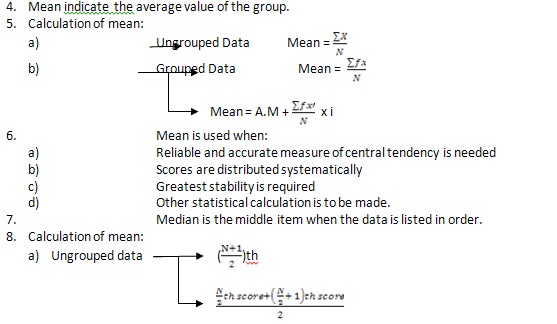6.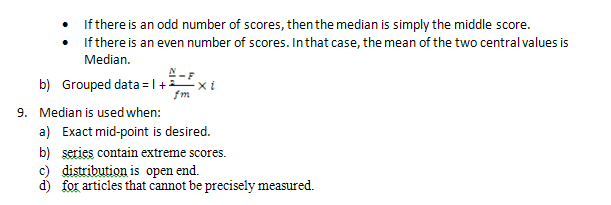Bibliography

1.      Biswal, B. Stastics in Education & Psychology, Dominant Publishers and Distributers,
New Delhi, 2006
2.      Text Book of Mathematics for class 10th, KPK text book board Peshawar.